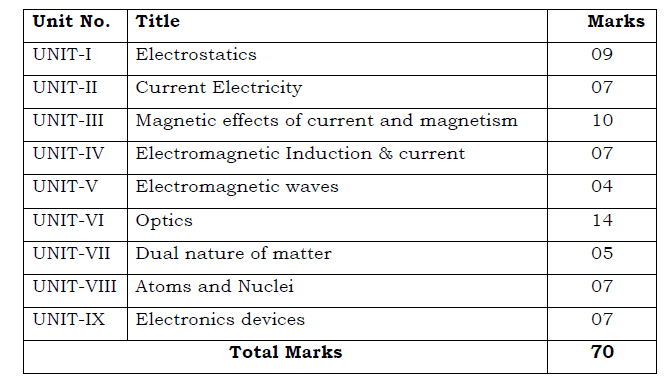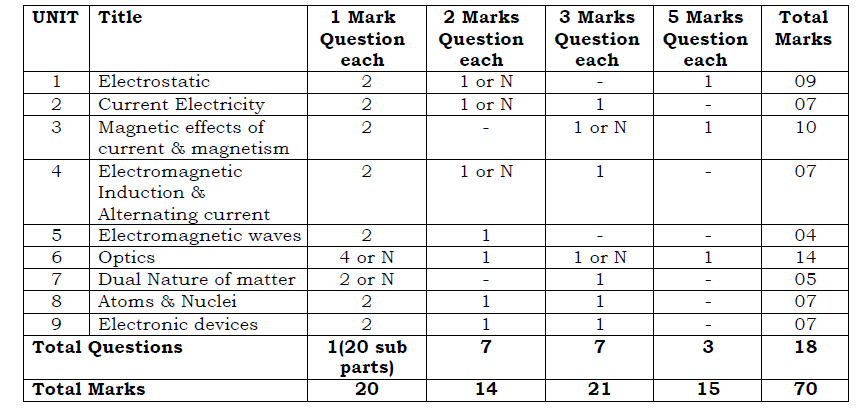# Punjab Board Class 12 Physics Syllabus

Since its inception, the Punjab School Education Board has been striving to provide world-class education in Science. By adopting novel methods such as introducing major scientific developments in the syllabus and following the guidelines laid down by the National Curriculum Framework 2005, the board has set a credible PSEB Class 12 Physics syllabus that puts the students on a path towards higher education.

The Punjab Board Class 12 Physics Syllabus has all the required components for a great foundation before the students can choose engineering or other academic courses. The board has adopted a future focus curriculum so that the topics are included in the syllabus before they are in demand in the industry.

From the links given below in this article. students can access the PDF version of the PSEB Class 12 Physics Syllabus 2021-22. We have also provided the snapshot of the unit wise marks distribution of the previous year.

Physics subject is not complete if experiments and practicals don’t go hand in hand with theory classes. To this extent, the Punjab board has included a right mix of practicals in the syllabus, so the students can also gain application perspective.

### Unitwise Marks Distribution based on 2020-21 Exam Pattern### Schematic Distribution Of Marks For Physics Exam Paper 2020-21### PSEB Class 12 Physics Practical Syllabus

 Experiments SECTION-A 1. To determine resistance per unit length of a given wire by plotting a graph of potential difference versus current. 2. To find resistance of a given wire using meter bridge and hence determine the specific resistance of its material. 3. To verify the laws of combination (series/parallel) of resistance using a meter bridge. 4. To compare the emf of two given primary cells using potentiometer. 5. To determine the internal resistance of given primary cell using potentiometer. 6. To determine the resistance of a galvanometer by half-deflection method and to find its figure of merit 7. To convert the given galvanometer of known resistance and figure of merit into an ammeter and voltmeter of desired range and to verify the same. 8. To find the frequency of the A.C. mains using a sonometer and electromagnet. SECTION-B 1. To find the value of v for different values of u in case of a concave mirror and find their focal length. 2. To find the focal length of a convex lens by plotting graphs between u and v or between 1/u and I/v. 3. To find the local length of a convex mirror, using a convex lens. 4. To find the focal length of a concave lens, using a convex lens. 5. To determine the angle of minimum deviation for a given prism by plotting a graph between angle of incidence and angle of deviation. 6. To draw the I-V characteristic curve of a p-n junction in forward bias and reverse bias. 7. To draw the characteristic curve of a zener diode and to determine its reverse breakdown voltage. 8. To study the characteristics of a common-emitter npn or pnp transistor and to find out the value of current and voltage gains. 9. To determine the reflective index of a glass slab using a traveling microscope. 10. To find the refractive index of a liquid by using (i) Concave mirror. (ii) Convex lens and plane mirror.

ACTIVITIES
SECTION-A
1. To assemble the components of a given electrical circuit.
2. To draw the diagram of a given open circuit comprising at least a battery, resistor Rheostat, key ammeter and volt meter. Mark the components that are not connected in proper order and correct the circuit and also the circuit diagram.
3. To assemble a household circuit comprising three, bulbs, three (on/off) switches, a – fuse and a power source.
4. To study the variation in potential drop with length of a wire for a steady current.
5. To measure resistance, voltage (AC/DC), current (AC) and check continuity of a given circuit using multimeter.
6. To measure the resistance and impedance of an inductor with or without iron core.
7. To demonstrate
(i) The use of an improvised fuse that melts with the flow of a certain current
through it and
(ii) Different kinds of fuses used in everyday life.
8. To demonstrate that a current measuring device has finite non- zero resistance(measurement of resistance of an ammeter).
9. To demonstrate that a voltage measuring device has non- infinite resistance(measurement of resistance of a voltmeter).
10. To show that earth’s magnetic field has both vertical & horizontal components,by using dip needle.
11. To show the magnetic field lines with the help of iron fillings of bar magnet solenoid.
12. To show the production of induced emf. in a coil due to movement of (i) a magnet towards and away from it (ii) similar coil carrying current towards & away from it.
13. To show that there are two kinds of charges and that like charges repel and unlike charges attract each other.
14. To demonstrate that a large emf is induced when direct current is switched off in an inductive circuit.
15. Make a solenoid for study of its magnetic field.

SECTION-B

1. To identify a diode, an LED, a transistor and 1C, a resistor and a capacity from mixed collection of such items.
2. Use of multimeter to (i) identify base of transistor, (ii) distinguish between npn ad pnp type transistors, (iii) see the unidirectional flow of current in case of a diode and an LED. iv) Check whether a given electronic component (e.g. diode, transistor or IC) is in working order.
3. To observe refraction and lateral deviation of a beam of light incident obliquely on a glass stab.
4. To study the nature arid size of the image formed by (i) convex lens (ii) concave mirror, on a screen by using a candle and a screen (for different distances of the candle from the lens/mirror).
5. To obtain a lens combination with the specified focal length by using two lenses from the given set of lenses.
6. To observe polarization of light using two Polaroids.
7. To observe diffraction of light due to a thin slit.
8. To study the effect of intensity of light (by varying distance of the source) on an D.R.

For more details or notifications about Punjab Board Class 12 , students can reach out to BYJU’S. We also offer pseb 12th question paper 2017